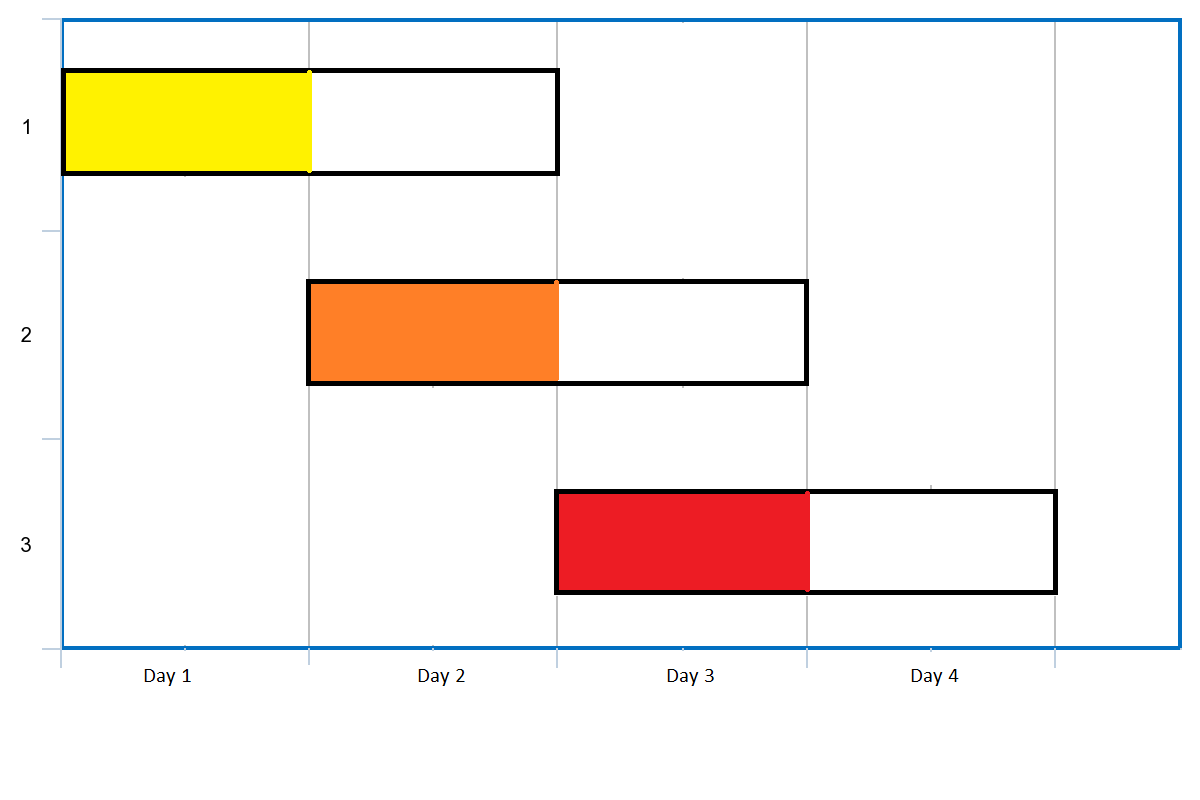## 274. H指数

h 指数的定义: “h 代表“高引用次数”（high citations），一名科研人员的 h 指数是指他（她）的 （N 篇论文中）至多有 h 篇论文分别被引用了至少 h 次。（其余的 N - h 篇论文每篇被引用次数不多于 h 次。）”

## 621. 任务调度器

• 任务的总个数为 [1, 10000]
• n 的取值范围为 [0, 100]

## 763. 划分字母区间

Difficulty: 中等

• S的长度在[1, 500]之间。
• S只包含小写字母 'a''z'

## 435. 无重叠区间

• 可以认为区间的终点总是大于它的起点。
• 区间 [1,2] 和 [2,3] 的边界相互“接触”，但没有相互重叠。

171ms，8%，感觉快要过不了了。。。本来是是写的记忆化递归的，结果过不了。。。卡在倒数第二个case上

## 1288. 删除被覆盖区间

Difficulty: 中等

• 1 <= intervals.length <= 1000
• 0 <= intervals[i] < intervals[i] <= 10^5
• 对于所有的 i != jintervals[i] != intervals[j]

Difficulty: 中等

Example:

## 1024. 视频拼接

• 1 <= clips.length <= 100
• 0 <= clips[i], clips[i] <= 100
• 0 <= T <= 100

## 1262. 可被三整除的最大和

• 1 <= nums.length <= 4 * 10^4
• 1 <= nums[i] <= 10^4

O(NlogN)贪心，最优解法应该是dp，放在dp专题中

## 5172. 形成三的最大倍数

• 1 <= digits.length <= 10^4
• 0 <= digits[i] <= 9
• 返回的结果不应包含不必要的前导零。

177周赛的T4，时隔多日，周赛又出了这一题，和上面一样，思路差不多的，需要优先考虑只删除一个的情况

## 1353. 最多可以参加的会议数目• 1 <= events.length <= 10^5
• events[i].length == 2
• 1 <= events[i] <= events[i] <= 10^5

## 134. 加油站

• 如果题目有解，该答案即为唯一答案。
• 输入数组均为非空数组，且长度相同。
• 输入数组中的元素均为非负数。

## 1046. 最后一块石头的重量

• 如果 x == y，那么两块石头都会被完全粉碎；
• 如果 x != y，那么重量为 x 的石头将会完全粉碎，而重量为 y 的石头新重量为 y-x

1. 1 <= stones.length <= 30
2. 1 <= stones[i] <= 1000

## 920. 会议室(LintCode)

• (0,8),(8,10)在8这这一时刻不冲突

## 919. 会议室 II(LintCode)## NC531.递增数组

• 1 <= array.size <= 2*10^5
• 1 <= array[i] <= 1*10^9

1. i后面的部分是单调递增的 3 1(i) 2 3，那么很明显这里和后面一起增加是最优选择
2. i后面是部分是单调递减的 3 3(i) 2 1，那么同样，和后面的一起增加是最优选择，单独选择某一个区间都会导致整体的落差变大，使得后面没增加的部分需要增加的次数增加
3. i后面先递增后递减 3 1(i) 2 1 同上 相当于 递增+递减 看做两部分，（1 2）同增，那么（2，1）也应该随之同增
4. i后面先递减后递增 5 4(i) 3 5 递减+递增 也分成两部分

## 738. 单调递增的数字

Difficulty: 中等

（当且仅当每个相邻位数上的数字&nbsp;x&nbsp;和&nbsp;y&nbsp;满足&nbsp;x &lt;= y&nbsp;时，我们称这个整数是单调递增的。）

（当且仅当每个相邻位数上的数字 x 和 y 满足 x <= y 时，我们称这个整数是单调递增的。）